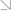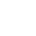全国热线：400-963-0708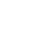网站地图 Sign in Sign up

# FRM考试科目定量分析的主题和阅读的相关内容是什么？

2021年新版FRM备考资料下载
• 考纲对比
• 学习计划
• 思维导图
• 复习资料
• 历年真题
• 词典及公式FRM考试中定量分析（Quantitative Analysis）所占比重是20%，因此在考试中考生需要对相关的内容有所了解。

This area tests a candidate’s knowledge of basic probability and statistics, regression and time series analysis, and various quantitative techniques useful in risk management. The broad knowledge points covered in Quantitative Analysis include the following:》》》2021年新版FRM一二级内部资料免费领取！【精华版】

• Discrete and continuous probability distributions

• Estimating the parameters of distributions

• Population and sample statistics

• Bayesian analysis

• Statistical inference and hypothesis testing• Measures of correlation

• Linear regression with single and multiple regressors

• Time series analysis and forecasting

• Simulation methods 》》》FRM复习资料点我咨询

To cover these broad knowledge points, a new proprietary book has been created exclusively for FRM candidates. While detailed learning objectives associated with these readings are presented in the 2021 FRM Learning Objectives document, a brief summary of how to relate these readings to the knowledge points follows.

Chapters 1 through 6 introduce fundamental concepts related to probability, statistics, probability distributions,Bayesian analysis, hypothesis testing, and confidence intervals. Regression analysis is an important statistical tool used to investigate relationships between variables. Chapters 7 and 8 give a general introduction to single and multiple variable linear regression analysis. Chapter 9 examines model specification and potential deficiencies in model specification through the use of residual diagnostics and tests of statistical hypotheses.【资料下载】[融跃财经]FRM一级ya题-pdf版

Time series data occur frequently in finance. The next two chapters describe methods for analyzing time series data in order to estimate statistics and extract other meaningful data characteristics. Chapter 10 focuses on modeling stationary time series, while Chapter 11 focuses on modeling nonstationary time series. Dependence and variation are important subjects in risk management. Chapter 12 introduces volatility, correlation, and returns, as well as the properties of these three measures in the context of both normally and non-normally distributed variables.

Simulation methods are used to value and analyze complex financial instruments and portfolios. Chapter 13 introduces simulation methods, including Monte Carlo simulation, and the use of bootstrapping. It also explains the advantages and disadvantages of using simulations and the techniques to reduce Monte Carlo sampling error.FRM学习交流群(扫码免费加群)

•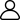1、新手入门
•2、学习资料
•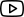3、免费课程
•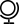4、考试动态
•5、备考干货
•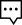6、答疑冲刺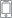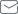2021年7月10日 FRM考试 还有

100
10小时 10分钟 10

## 推荐课程